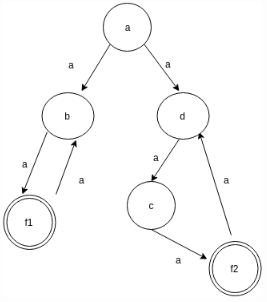Courses

# Test: Epsilon Closures

## 8 Questions MCQ Test Theory of Computation | Test: Epsilon Closures

Description
This mock test of Test: Epsilon Closures for Computer Science Engineering (CSE) helps you for every Computer Science Engineering (CSE) entrance exam. This contains 8 Multiple Choice Questions for Computer Science Engineering (CSE) Test: Epsilon Closures (mcq) to study with solutions a complete question bank. The solved questions answers in this Test: Epsilon Closures quiz give you a good mix of easy questions and tough questions. Computer Science Engineering (CSE) students definitely take this Test: Epsilon Closures exercise for a better result in the exam. You can find other Test: Epsilon Closures extra questions, long questions & short questions for Computer Science Engineering (CSE) on EduRev as well by searching above.
QUESTION: 1

### Which of the following does not belong to input alphabet if S={a, b}* for any language?

Solution:

The automaton may be allowed to change its state without reading the input symbol using epsilon but this does not mean that epsilon has become an input symbol. On the contrary, one assumes that the symbol epsilon does not belong to any alphabet.

QUESTION: 2

### The number of final states we need as per the given language?Language L: {an| n is even or divisible by 3}

Solution: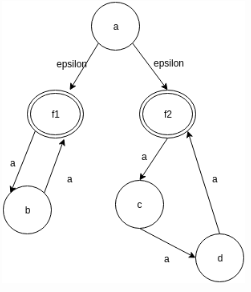QUESTION: 3

### State true or false:Statement: Both NFA and e-NFA recognize exactly the same languages.

Solution:

e-NFA do come up with a convenient feature but nothing new.They do not extend the class of languages that can be represented.

QUESTION: 4

Design a NFA for the language:
L: {an| n is even or divisible by 3}
Which of the following methods can be used to simulate the same.

Solution:

It is more convenient to simulate a machine using e-NFA else the method of Power Construction is used from the union-closure of DFA’s.

QUESTION: 5

Which of the following belongs to the epsilon closure set of a?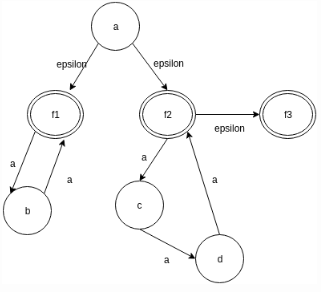Solution:

The epsilon closure of the set q is the set that contains q, together with all the states which can be reached starting at q by following only epsilon transitions.

QUESTION: 6

The number of elements present in the e-closure(f2) in the given diagram: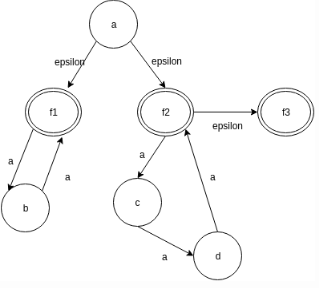Solution:

The epsilon closure set of f2 consist of the elements:{f2, f3}. Thus the count of the element in the closure set is 2.

QUESTION: 7

Which of the steps are non useful while eliminating the e-transitions for the given diagram?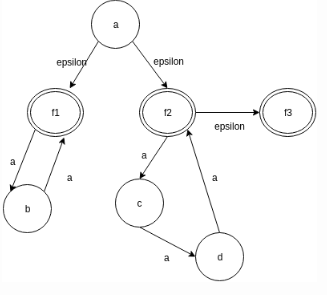Solution:

The given are the steps followed while eliminating epsilon transitions from a NFA or converting an e-NFA to just NFA.

QUESTION: 8

Remove all the epsilon transitions in the given diagram and compute the number of a-transitions in the result?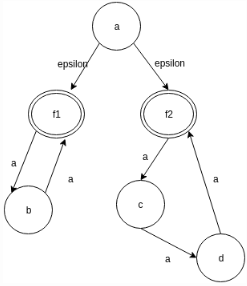Solution: